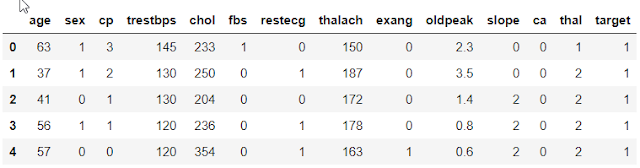## InfinityCodeX

Check out our blogs where we cover topics such as Python, Data Science, Machine Learning, Deep Learning. A Best place to start your AI career for beginner, intermediate peoples.

# How To Find Optimal Threshold Value And Change Threshold Value In Logistic Regression

Whenever we learn Logistic Regression, we always encounter the Question that How to find the optimal Threshold value for our model? or How we can change the Threshold value as per user’s requirement?

If someone who doesn’t know what is a Threshold value in Logistic Regression then you must check out this link

https://www.infinitycodex.in/logistic-regression-in-machine-learning

And for those who know what threshold value is can keep on reading.

Now why some users/client want to change there threshold value & at which problem we should decide that we have to change the threshold value.

Now the threshold value can be increased or decreased based on the problem or dataset we are dealing with. By the way, the default threshold value in Logistic Regression is 0.5. Now you must be thinking why the hell we want to increase or decrease the threshold value?

Let’s understand this with 2 simple scenarios one for decreasing and others for increasing the threshold value.

1.) Decreasing the Threshold value:

Let’s say you want to predict that the students who score less than 30% failed in there examination. So at this problem statement, you have to reduce the threshold value at 30%.

2.) Increasing the Threshold value:

Let’s say you got a cancer dataset and doctor’s told you that you have to predict the person is having cancer or not. If the person is above 80% infected than that person should be considered as worst infected by cancer and need immediate chemotherapy if that person is having less than 80% the chance that we can consider that person can be treated with normal medication.

I know most of them to know all this thing already by the main question is how do we code this entire process from finding optimal Threshold value till the changing the threshold value.

We will be using the ROC Curve which will help us to predict the optimal threshold value. For those who don't know what the ROC Curve is... ROC Curve is known as Receiver Operating Characteristic.

* ROC Curve is used in Binary Classification.

* It is a plot of True Positive Rate(1) on Y-Axis against False Positive Rate(0) on X-Axis.

* Once you get the output in probability. You can use the different cut-off to distinguish what is going to be the True Case and what will be the False Case.

* ROC Curve say when your curve is closer to the Y-Axis that is True Positive Rate than it is a very good model and your model is in between that is 0.5 than it's an average model and if your curve is towards the False Positive Rate than it's the worst model.

ROC Curve looks something like this:

CODE:

import pandas as pd
import numpy as npdata = pd.get_dummies(data, columns=['cp','slope','thal','restecg'], drop_first=True)

from sklearn.model_selection import train_test_split

X = data.drop('target', axis=1).values
y = data['target'].values

X_train, X_test, y_train, y_test = train_test_split(X, y, test_size=0.2, random_state=42)

Normalizing the values using MinMaxScaler.

from sklearn.preprocessing import MinMaxScaler

scaler = MinMaxScaler()

X_train = scaler.fit_transform(X_train)
X_test = scaler.transform(X_test)

Find Threshold Value

Here you can use Decision Tree, Random Forest, Bagging, Boosting.. etc.

from sklearn.metrics import roc_auc_score, roc_curve

# Random Forest
from sklearn.ensemble import RandomForestClassifier

rf_model = RandomForestClassifier()
rf_model.fit(X_train, y_train)

rf_ytrain_pred = rf_model.predict_proba(X_train)
print("RF Train roc-auc:{}".format(roc_auc_score(y_train, rf_ytrain_pred[:,1])))

rf_y_test_pred = rf_model.predict_proba(X_test)
print("RF Test roc-acc:{}".format(roc_auc_score(y_test, rf_y_test_pred[:,1])))

#----------------------------------------------------------------------------------

# Logistic Regression
from sklearn.linear_model import LogisticRegression

lg_model = LogisticRegression()
lg_model.fit(X_train, y_train)

lg_ytrain_pred = lg_model.predict_proba(X_train)
print("LG Train roc-auc:{}".format(roc_auc_score(y_train, lg_ytrain_pred[:,1])))

lg_y_test_pred = lg_model.predict_proba(X_test)
print("LG Test roc-auc:{}".format(roc_auc_score(y_test, lg_y_test_pred[:,1])))

Output:

RF Train roc-auc:1.0
RF Test roc-acc:0.915948275862069
LG Train roc-auc:0.9226736566186108
LG Test roc-auc:0.9450431034482759

Selection Of Best Threshold Value For Accuracy

pred = []

for model in [rf_model, lg_model]:
pred.append(pd.Series(model.predict_proba(X_test)[:,1]))

final_pred = pd.concat(pred, axis=1).mean(axis=1)
print("Ensemble test roc-auc:{}".format(roc_auc_score(y_test,final_pred)))

Output:

Ensemble test roc-auc:0.9407327586206897

# Calculate the roc-curve

False_pos_rate, True_pos_rate, threshold = roc_curve(y_test, final_pred)

threshold

Output:

array([1.97525308, 0.97525308, 0.82803343, 0.82740393, 0.61206134, 0.5829135 , 0.48185878, 0.45554149, 0.45229139, 0.38156861, 0.21880427, 0.07000419, 0.06909258, 0.0069847 ])

These are some of the best candidates from which we can select our threshold value.

from sklearn.metrics import accuracy_score

acc = []

for thres in threshold:
y_pred = np.where(final_pred>thres,1,0)

#what ever prediction i am getting and if it is greater than threshold i'll be converting as 1 or i'll keep it as 0.

acc.append(accuracy_score(y_test,y_pred,normalize=True))

#Then i'll be computing my accuracy score with my y_test and then
#append the accuracy inside acc list.

acc = pd.concat([pd.Series(threshold), pd.Series(acc)], axis=1)
acc.columns = ['threshold','accuracy']
acc.sort_values(by="accuracy", ascending=False, inplace = True)

These are top 5 threshold values.

import matplotlib.pyplot as plt
def plot_roc_curve(False_pos_rate,True_pos_rate):
plt.plot(False_pos_rate, True_pos_rate, label="ROC")
plt.plot([0,1],[0,1],color="Red",linestyle="--")
plt.xlabel("False_Positive_rate")
plt.ylabel("True_Positive_rate")
plt.title("ROC Curve")
plt.legend
plt.show()

plot_roc_curve(False_pos_rate,True_pos_rate)

These are some of the best candidates from which we can select our threshold value.

Create A Model with 0.61 Threshold

from sklearn.linear_model import LogisticRegression
from sklearn.metrics import accuracy_score, confusion_matrix, recall_score, roc_auc_score, precision_score, classification_report

clf = LogisticRegression(class_weight="balanced")
clf.fit(X_train, y_train)

THRESHOLD = 0.61
preds = np.where(clf.predict_proba(X_test)[:,1] > THRESHOLD, 1, 0)

pd.DataFrame(data=[accuracy_score(y_test, preds), recall_score(y_test, preds), precision_score(y_test, preds), roc_auc_score(y_test, preds)], index=["accuracy", "recall", "precision", "roc_auc_score"])

A model with Changed threshold value

print(classification_report(y_test,preds))

print(confusion_matrix(y_test,preds))

[[28 1]
[ 9 23]]

So we hope that you find the solution. If you found this helpful then please share it with your friends and spread this knowledge.

Instagram :

https://www.instagram.com/infinitycode_x/

1.